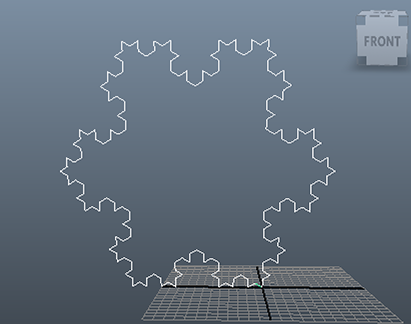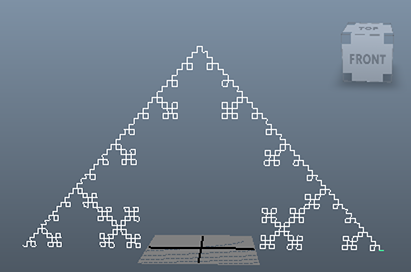### MelBarebones L-System

#### Introduction

This tutorial presents a simple L-System intended to create a Koch snowflake, figure 1, and similar shapes (figure 2). Despite Mel being somewhat clumsy in regard to the handling of strings the code presented in listing 1 does demonstrate the principles of L-System string re-writing and string interpretation. A more extensive python implementation of a L-System can be found in the tutorial "L system: Code and Testing".Figure 1
\$axiom = "L<zzL<zzL";
\$ruleL = "L>zL<zzL>zL";
\$angle = 60.0;Figure 2
\$axiom = ">zL";
\$ruleL = "L<zL>zL>zL<zL";
\$angle = 90.0;

##### The Scripts

The mel scripts in listings 1, 2 and 3 should be saved in the "scripts" directory of a Maya project directory. For example,

```    maya/projects/Koch/scripts/koch.mel
maya/projects/Koch/scripts/node_utils.mel
maya/projects/Koch/scripts/preamble.mel```

When the main script, kock.mel, is run it will generate a script named "snowflake.mel" that when sourced,
```    rehash;     source "snowflake.mel"; ``` will generate the shape shown in figure 1. Interpreting a string of characters as a sequence mel transformations and geometries is a little tricky. For example,
`zL`
means "apply a rotation around the z axis", then "insert a straight line curve" ie.

```    rotate 0 0 60.0;
curve -d 1 -p 0 0 0 -p 0 1 0;```

In mel, however, the rotate statement is normally specified AFTER the geometry ie.

```    curve -d 1 -p 0 0 0 -p 0 1 0;
rotate 0 0 60.0;```

otherwise the transformation will not effect the curve! The "node_utils.mel" (listing 2) and "preamble.mel" (listing 3) ensure that transformations and geometries are "parented" to a group node named "\$tnode". The parenting enables the characters of a L strings to be interpreted in the classic "transform followed geometry" sequence without the need to rearrange the characters in either the "axiom" or the "rule". This makes it relatively easy to adapt examples of L strings taken from texts that deal with "Algorithmic Botany".

#### Extension to 3D

If the `convertToMel()` proc (koch.mel) is extended to handle rotations around the x and y axes some interesting 3D forms can be generated. 3D extension are left to the reader to explore.Figure 3 \$axiom = ">zL"; \$ruleL = ">yLxL>zL>zL>xL

Listing 1 (koch.mel)

 ```/* A barebones example of an L-System for creating a Koch snowflake fractal. Save this script, "node_utils.mel" and "preamble.mel" in the scripts folder of the Maya project directory that is used for visualizing the graphics output. Started: Jan 9 2015 Malcolm Kesson Character Interpretation: L draw a (stright curve) line < subsequent rotations are positive > subsequent rotations are negative x rotation axis is 'x' y rotation axis is 'y' z rotation axis is 'z' */ global float \$angle = 60.0; global string \$ruleL = "L>zLzL"; string \$axiom = "L". global proc string convertToMel(string \$lstring, string \$name) { global float \$angle; string \$lines[]; int \$line_count = 0; int \$n; for(\$n = 0; \$n < size(\$lstring); \$n++) { string \$c = substring(\$lstring, \$n+1, \$n+1); if(\$c == "<") \$angle = abs(\$angle); else if(\$c == ">") \$angle = abs(\$angle) * -1; else if(\$c == "L") \$lines[\$line_count++] = "\$tnode = addCurveTo(\$tnode);\n"; else if(\$c == "z") { //\$angle = rand(\$angle - 5, \$angle + 5); \$lines[\$line_count++] = "rotate -r 0 0 " + \$angle + " \$tnode;\n"; } } // In addition to generating an output "snowflake.mel" data script // we make use of two other mel scripts. The "utilities.mel" script // is sourced by "snowflake.mel". The contents of the "preamble.mel" // script is read and added to "snowflake.mel". Finally, the mel // statements generated from the Lstr are added to "snowflake.mel". string \$projPath = `workspace -q -rootDirectory`; string \$scriptsPath = \$projPath + "scripts/"; // 1. Open the output "Koch_shape.mel" for writing. string \$output_path = \$scriptsPath + \$name; int \$Koch_output = fopen(\$output_path, "w"); // 2. Add the source statement for the node utilities mel script. string \$source = "source \"" + \$scriptsPath + "node_utils.mel\";\n"; fprint(\$Koch_output, \$source); // 3. Read "preamble.mel" and add its statements. int \$preamble = fopen(\$scriptsPath + "preamble.mel", "r"); string \$text; \$text = fread(\$preamble, \$text); fprint(\$Koch_output, \$text); fclose(\$preamble); // 4. Finally, write the mel commands that define the shape of // the Koch snowflake. for(\$n = 0; \$n < size(\$lines); \$n++) fprint(\$Koch_output, \$lines[\$n]); fclose(\$Koch_output); return \$output_path; } string \$Lstr = rewrite(\$axiom, 4); string \$outpath = convertToMel(\$Lstr, "snowflake.mel"); // print(\$Lstr + "\n"); print("Final LString has " + size(\$Lstr) + " characters.\n");```

Listing 2 (node_utils.mel)

 ```//Author: Malcolm Kesson (2007) string \$stack[]; int \$index = 0; //=============================================== // addCurveTo //=============================================== // Attaches a curve to the input group "node" then // creates an empty group and makes it a child of // the curve (transformation) node. So that the // next curve will "grow" from the end of the current // curve the local coordinate system is moved up // one unit. global proc string addCurveTo(string \$node) { \$shape = `curve -d 1 -p 0 0 0 -p 0 1 0`; parent -r \$shape \$node; \$child = `group -em`; parent -r \$child \$shape; move -os 0 1 0 \$child; return \$child; } global proc string addConeTo(string \$node) { \$shape = `cone -ax 0 1 0 -r 1 -hr 3`; parent -r \$shape \$node; \$child = `group -em`; parent -r \$child \$shape; move -os 0 3 0 \$child; return \$child; } global proc string addShapeTo(string \$node, string \$shapeCmd, float \$premove, float \$postmove) { \$shape = eval(\$shapeCmd); move -os 0 \$premove 0; parent -r \$shape \$node; \$child = `group -em`; parent -r \$child \$shape; move -os 0 \$postmove 0 \$child; return \$child; } //=============================================== // push - transformation stack //=============================================== // To enable branching to occur we must keep a // reference to the "active" group node so that // we can return to it for parenting. global proc string push(string \$node) { global string \$stack[]; global int \$index; \$child = `group -em`; \$test = `duplicate \$node`; \$stack[\$index] = \$test; \$index += 1; return \$child; } //=============================================== // pop - transformation stack //=============================================== // Calling this proc enables us to return to the // base of a branch. global proc string pop() { global string \$stack[]; global int \$index; \$index -= 1; if(\$index < 0) { print("Error: stack has become negative\n"); return ""; } return \$stack[\$index]; }```

Listing 3 (preamble.mel)

 ```global string \$stack[]; \$nulls = `ls -tr "LSYS"`; if(size(\$nulls) > 0) { select \$nulls; delete; } \$geoList = `ls -geometry`; select \$geoList; delete; \$nulls = `ls -tr "null*"`; select \$nulls; delete; clear(\$stack); \$root = `group -em -n LSYS`; \$tnode = `group -em`; parent -r \$tnode \$root;```